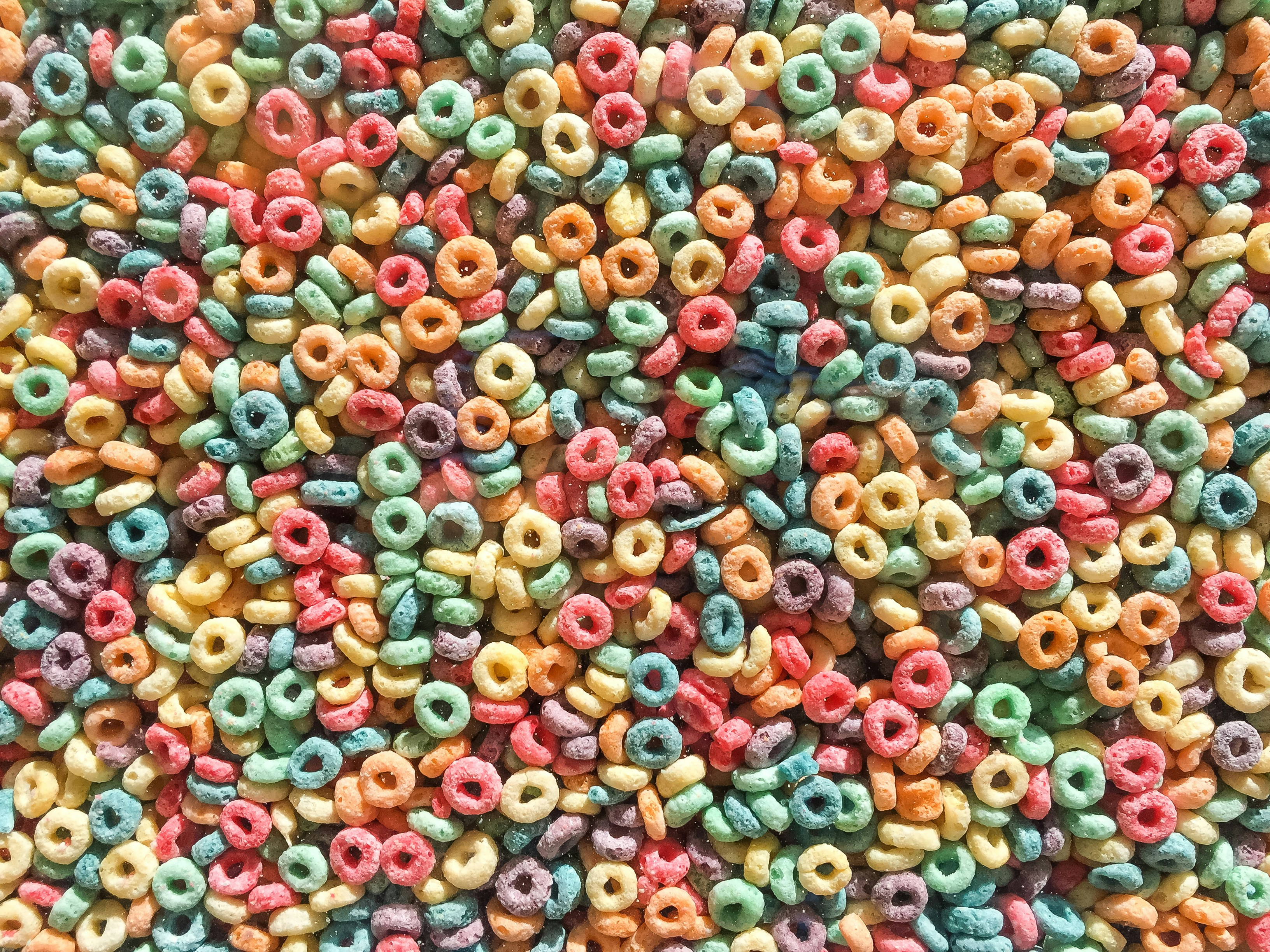# Mastering Go For Loops - A Comprehensive Guide

Posted on:October 8, 2023 at 11:40 PM# Introduction

### The Basic Syntax

The basic syntax of a for loop in Go is straightforward:

``````for initialization; condition; post {
// Code to be executed
}``````
• Initialization: This part is executed before the loop begins and typically initializes a variable that will be used in the loop.
• Condition: The loop continues executing as long as the condition is true.
• Post: This part is executed after each iteration of the loop and is often used to update the loop control variable.

Let’s break down each component with examples:

#### Initialization

In this section, you can declare and initialize variables that will be used within the loop. For instance:

``````for i := 0; i < 5; i++ {
fmt.Println(i)
}``````

In this example, `i` is initialized to 0, and the loop will continue as long as `i` is less than 5. After each iteration, `i` is incremented by 1.

#### Condition

The loop will continue executing as long as the condition is true. If the condition is false from the beginning, the loop won’t run at all. For example:

``````for j := 10; j > 0; j-- {
fmt.Println(j)
}``````

This loop counts down from 10 to 1. Once `j` becomes 0, the condition is false, and the loop terminates.

### Post

The post statement is executed after each iteration of the loop and is often used to update the loop control variable. Here’s an example:

``````for x := 0; x < 10; x += 2 {
fmt.Println(x)
}``````

In this case, `x` starts at 0 and is incremented by 2 after each iteration, producing the numbers 0, 2, 4, 6, and 8.

### Using For Loops

#### Looping Over Arrays and Slices

For loops are commonly used to iterate over arrays and slices in Go:

``````numbers := []int{1, 2, 3, 4, 5}

for index, value := range numbers {
fmt.Printf("Index: %d, Value: %d\n", index, value)
}``````

The `range` keyword is used to iterate over the elements of a slice or an array, providing both the index and the value of each element in each iteration.

#### Infinite Loops

In the world of go we don’t have a `while` loop; but we can create an infinite loop by omitting the initialization, condition, and post statements. Use caution when creating infinite loops, as they can lead to program hangs if not controlled properly. For example:

``````for {
// This is an infinite loop
}``````

### Using For Loops with Maps

In addition to iterating over arrays and slices, Go for loops are also useful for traversing the elements of a map. Here’s an example of how you can loop over a map and perform actions on its key-value pairs:

``````// Create a map with some key-value pairs
"Alice":   90,
"Bob":     85,
"Charlie": 78,
"David":   92,
}

// Loop over the map using the "range" keyword
}``````

In this example, we have a map called `studentGrades` that associates student names with their grades. We use a `for` loop with the `range` keyword to iterate over the map. During each iteration, the `student` variable holds the key (student name), and the `grade` variable holds the corresponding value (student’s grade).

The output of this code will be:

``````Student: Alice, Grade: 90
This example demonstrates how to effectively loop over a map in Go, allowing you to access and process key-value pairs within your programs. Whether you need to analyze data stored in maps or perform specific operations on map elements, the `for` loop combined with `range` provides a powerful and flexible way to work with maps in Go.